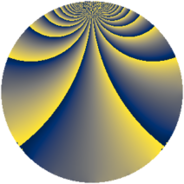# Properties

 Label 605.2.jLevel $605$ Weight $2$ Character orbit 605.j Rep. character $\chi_{605}(9,\cdot)$ Character field $\Q(\zeta_{10})$ Dimension $184$ Newform subspaces $11$ Sturm bound $132$ Trace bound $6$

# Related objects

## Defining parameters

 Level: $$N$$ $$=$$ $$605 = 5 \cdot 11^{2}$$ Weight: $$k$$ $$=$$ $$2$$ Character orbit: $$[\chi]$$ $$=$$ 605.j (of order $$10$$ and degree $$4$$) Character conductor: $$\operatorname{cond}(\chi)$$ $$=$$ $$55$$ Character field: $$\Q(\zeta_{10})$$ Newform subspaces: $$11$$ Sturm bound: $$132$$ Trace bound: $$6$$ Distinguishing $$T_p$$: $$2$$, $$19$$

## Dimensions

The following table gives the dimensions of various subspaces of $$M_{2}(605, [\chi])$$.

Total New Old
Modular forms 312 248 64
Cusp forms 216 184 32
Eisenstein series 96 64 32

## Trace form

 $$184 q + 44 q^{4} + 3 q^{5} + 18 q^{6} + 26 q^{9} + O(q^{10})$$ $$184 q + 44 q^{4} + 3 q^{5} + 18 q^{6} + 26 q^{9} + 16 q^{14} + 13 q^{15} - 40 q^{16} - 6 q^{19} + 4 q^{20} - 8 q^{21} - 6 q^{24} + 13 q^{25} - 84 q^{26} - 2 q^{29} - 26 q^{30} - 2 q^{31} - 80 q^{34} - 22 q^{35} - 2 q^{36} - 30 q^{39} - 12 q^{40} + 52 q^{41} - 84 q^{45} + 62 q^{46} - 28 q^{50} + 42 q^{51} + 40 q^{54} - 12 q^{56} + 4 q^{59} + 90 q^{60} + 40 q^{61} - 28 q^{64} + 40 q^{65} - 36 q^{69} + 18 q^{70} - 54 q^{71} - 48 q^{74} - 21 q^{75} - 56 q^{76} - 38 q^{79} + 16 q^{80} - 42 q^{81} - 12 q^{84} - 58 q^{85} - 6 q^{86} - 144 q^{89} - 78 q^{90} + 28 q^{91} - 14 q^{94} - 48 q^{95} + 86 q^{96} + O(q^{100})$$

## Decomposition of $$S_{2}^{\mathrm{new}}(605, [\chi])$$ into newform subspaces

Label Dim $A$ Field CM Traces $q$-expansion
$a_{2}$ $a_{3}$ $a_{5}$ $a_{7}$
605.2.j.a $8$ $4.831$ 8.0.484000000.6 $$\Q(\sqrt{-55})$$ $$0$$ $$0$$ $$-10$$ $$0$$ $$q+(\beta _{1}-\beta _{4})q^{2}+(1+\beta _{2}-3\beta _{3}+3\beta _{5}+\cdots)q^{4}+\cdots$$
605.2.j.b $8$ $4.831$ 8.0.228765625.1 $$\Q(\sqrt{-11})$$ $$0$$ $$0$$ $$-3$$ $$0$$ $$q+(-\beta _{4}-2\beta _{5})q^{3}+(-2+2\beta _{3}+2\beta _{4}+\cdots)q^{4}+\cdots$$
605.2.j.c $8$ $4.831$ 8.0.484000000.6 $$\Q(\sqrt{-55})$$ $$0$$ $$0$$ $$10$$ $$0$$ $$q+(\beta _{1}-\beta _{6})q^{2}+(-2\beta _{2}+2\beta _{3}-3\beta _{5}+\cdots)q^{4}+\cdots$$
605.2.j.d $16$ $4.831$ $$\mathbb{Q}[x]/(x^{16} - \cdots)$$ None $$0$$ $$0$$ $$-2$$ $$0$$ $$q+(\beta _{1}-\beta _{12}-\beta _{13})q^{2}+(-\beta _{5}-\beta _{13}+\cdots)q^{3}+\cdots$$
605.2.j.e $16$ $4.831$ 16.0.$$\cdots$$.7 None $$0$$ $$0$$ $$0$$ $$0$$ $$q+\beta _{12}q^{2}+\beta _{3}q^{3}-\beta _{10}q^{4}+(-\beta _{2}+\cdots)q^{5}+\cdots$$
605.2.j.f $16$ $4.831$ 16.0.$$\cdots$$.7 None $$0$$ $$0$$ $$0$$ $$0$$ $$q+\beta _{12}q^{2}-\beta _{3}q^{3}-\beta _{10}q^{4}+(-\beta _{2}+\cdots)q^{5}+\cdots$$
605.2.j.g $16$ $4.831$ $$\mathbb{Q}[x]/(x^{16} - \cdots)$$ None $$0$$ $$0$$ $$-2$$ $$0$$ $$q+\beta _{13}q^{2}+(-\beta _{1}-\beta _{2}+\beta _{5})q^{3}+(\beta _{3}+\cdots)q^{4}+\cdots$$
605.2.j.h $16$ $4.831$ $$\mathbb{Q}[x]/(x^{16} - \cdots)$$ None $$0$$ $$0$$ $$-2$$ $$0$$ $$q+\beta _{13}q^{2}+(\beta _{1}+\beta _{2}-\beta _{5})q^{3}+(\beta _{3}+\cdots)q^{4}+\cdots$$
605.2.j.i $16$ $4.831$ 16.0.$$\cdots$$.1 None $$0$$ $$0$$ $$3$$ $$0$$ $$q+(\beta _{1}+\beta _{4}+\beta _{5}-\beta _{8}+\beta _{11}-\beta _{13}+\cdots)q^{2}+\cdots$$
605.2.j.j $16$ $4.831$ 16.0.$$\cdots$$.1 None $$0$$ $$0$$ $$3$$ $$0$$ $$q+(\beta _{1}+\beta _{4}+\beta _{5}-\beta _{8}+\beta _{11}-\beta _{13}+\cdots)q^{2}+\cdots$$
605.2.j.k $48$ $4.831$ None $$0$$ $$0$$ $$6$$ $$0$$

## Decomposition of $$S_{2}^{\mathrm{old}}(605, [\chi])$$ into lower level spaces

$$S_{2}^{\mathrm{old}}(605, [\chi]) \cong$$ $$S_{2}^{\mathrm{new}}(55, [\chi])$$$$^{\oplus 2}$$